## Search This Blog

### 223 versus 351

We've gone through all 2048 musical scales (represented by distinct polygons of various degrees of n-gonicity ranging from 1 to 12) that can be squeezed out of a universe of twelve tones - or more accurately pitch classes (represented by an ordinary 12 hour clock face). We've shown, with visual aids in the form of animated gifs, that many of these scales are 'the same' and that the number of significantly different scales is only 351 - a rather lower number than 2048.

But what do we mean by significantly different, or the same? It's in the sense that - to take a common example known to every practising musician - the minor diatonic scale is the same as the major diatonic scale. This may seem a slightly bizarre thing to regard as 'the same' since it's commonly held that the whole point of the minor scale is to produce pieces of music distinct in 'mood' from pieces produced in the major scale. But when enumerating/listing scales, it's not any actual piece of music written in that scale which concerns us. It's simply the sequence of (in that case, 7) notes which comprise that scale.

Our practicing musician knows very well that seven consecutive white notes on the piano do double duty as an A minor scale and also as a C major scale. You just start on a different note, the so called tonic A or C. They also know that there are another 5 possible scales available with these same seven consecutive white notes since there are seven choices for your first note. Your tonic may be A, or B, or C, D, E, F or G.

This is how the number is reduced from 2048 to 351 - take any random binary number in the range 2048 to 4095 and rotate the bits in it to the left, always rotating enough bits in one go to ensure that the top bit remains set because all our 2048 scales include that tonic at 12 (zero) o'clock represented by the leftmost bit. For example, 101011011111 (decimal 2783) is a nine-note scale where the first two bits can be rotated left (and reappear as the least significant two bits on the right) to get us to 101101111110. Another two bit left rotation gets us to 110111111010. Next, a one bit left rotation to 101111110101, a further two to 111111010110. That's four of the nine rotations of what we are calling 'the same' scale pattern. Then, a further five 1 bit left rotations take us through, successively, 111110101101, 111101011011, 111010110111, 110101101111 and finally 101011011111, ending up where we began. The corresponding polygons are shown below: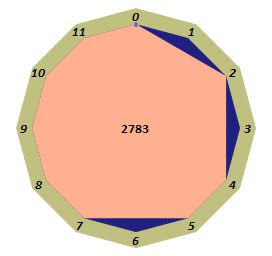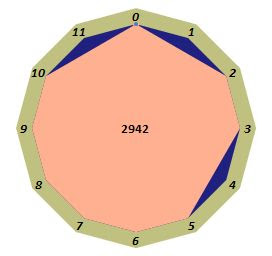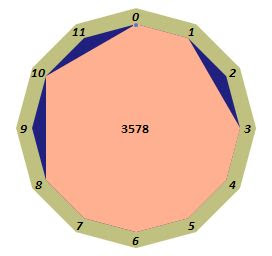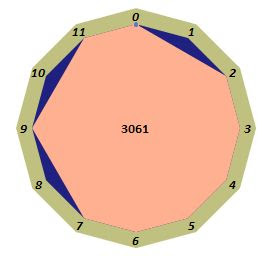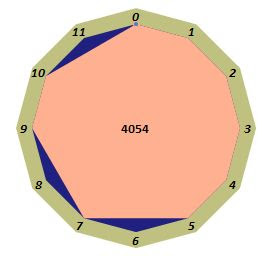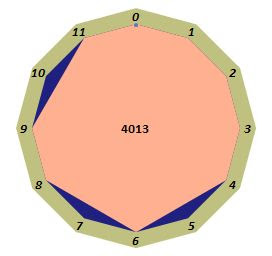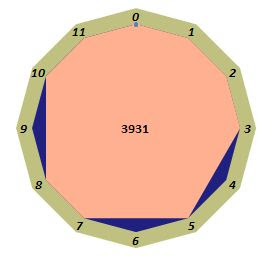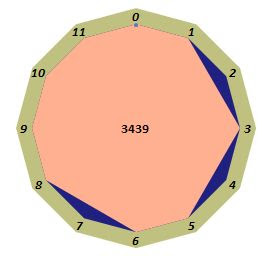As rotated polygons are regarded as being representative of the same scale, where the differing rotations are re-termed as 'modes' of that same scale (cf Aeolian, Dorian, Phrygian, modes etc when talking about the diatonic scale), it should be reasonably obvious by now that by 'equalising' bunches of polygons ten at a time, nine at a time, eight at a time etc, 2048 reduces quite quickly.

But we're promising to get that number down still further to 223. How do we achieve that? It's to do with inversion or, strictly speaking, the impossibility of scale-preserving inversion.

Something that practicing pianists will know about their instrument is that it is symmetric about the D key (next to middle C) and that therefore it's possible to perform true diatonic melodic inversions of motifs, melodic fragments, tunes (call them what you will) written in any of the seven modes of diatonicity. This is discussed in more detail in a post over here. Pianos are interesting things. Their peculiar but periodic arrangement of keys reveals so much that stringy, windy and bangy instruments hide. But one of the less obvious things is that scale-preserving inversions (by which we mean melodic inversions of a sequence of pitches in a chosen scale/mode (containing only those pitches within it) can be performed only within scales representable by symmetric polygons. If your scale polygon has no symmetry (such as our nonatonic example above) then - no matter how hard you try - you cannot invert a phrase written in that scale without introducing pitches not present in that scale (see e.g. our Hungarian illustration).

When considering chromatic inversion, however, it is always possible to invert a melodic line simply because - whatever scale you choose for your original phrase, i.e. howsoever you elect to limit the pitch classes within it - an inversion is always possible simply because you cannot emit (by that chromatic inversion) a pitch class outside of the chromatic (i.e. full dodecatonic) scale (with its one and only 'mode'). Since (chromatic) inversion is the (in our view, unfortunately lackadaisical) default interpretation of the term 'inversion', then it is permissible to also regard the many unsymmetric 'mirror image' scales as being equivalent to each other.

Note that it's not that we object to the allowance of dihedral symmetry as an equivalence relation. These polygons actually do reflect each other, they are very real and eminently accountable. It's the use of the term 'inversion' to include non-scale-preserving transformations we find a little too lax for our mathematical tastes. In Mathemania, inversion has a very much stricter group preserving attribute than that found in Musiconia. In formal (musical) set (another problematic-to-mathematicians term) theory there's no problem at all with the term 'inversion' since that theory concerns itself only with the full twelve tone scale.

What's the 'mirror' of scale 2783? We just flip its polygon around the 12 o'clock-six o'clock axis. In terms of the 12 bit representation this simply means keeping bits 0 and 6 fixed and swapping bits 1 and 11, 2 and 10, 3 and 9, 4 and 8, and 5 and 7. Thus (1)01011(0)11111 → (1)11111(0)11010 (scale 4058).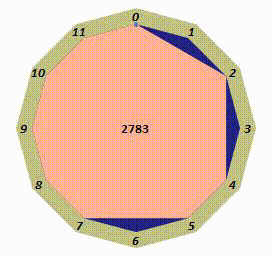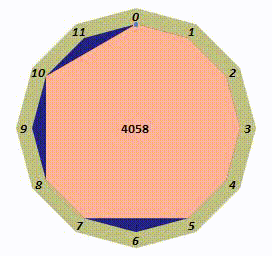Another, non-symmetric, nonatonic scale is 3023 (1)01111(0)01111 which mirrors scale 3998 (1)11110(0)11110. The animated gifs here show their nine rotations (i.e. their modes) each of which perforce preserves their particular modal mirroring.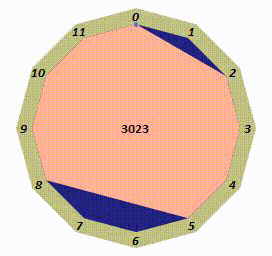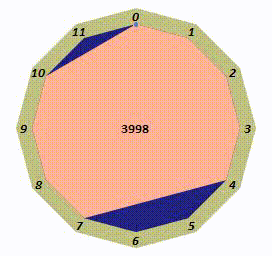Note that whilst it is impossible to do scale (as opposed to the always possible chromatic) inversions with these nonsymmetric scales, the attempt to do so (basically by negating, modulo 12, every pitch within the melodic line in the chosen scale) will result in a phrase consistent with its 'mirror' scale. That this must be the case is reasonably obvious since the pitch negative of 1 is 11, of 2 is 10, etc - the very operation we performed to reach our mirror polygon (i.e. by reflecting the 'hours' on our clock across the twelve-six axis - which is what subtraction from zero is).

This means it's possible to perform an inversion from one scale into its mirror scale, then transpose that new phrase whilst in the mirror scale, invert again and end up back in your original scale, but transposed (or - alternatively - in a different mode of the original key, it depends how you look at it). It's an interesting way of achieving modulations. Contrast this technique with scale inversions in scales representable by symmetric polygons. Whilst always possible, you forego the excitement of leaving the scale.

So far, then, we can extend the table (found at the end of our previous excursion into scale enumerations) by splitting that original bottom row (summing to 351) into the counting of what might be considered symmetric (invertible) and non-symmetric (uninvertible) scales.

Number of notes in scale
123456789101112total
11155165330462 46233016555111 2048
1619436680 664319611 351
symmetric:=
165151020 10155611 95
non-symmetric pairs: (i.e. to be doubled in the total)+
007142830 28147000 256
95 invertible + 128 uninvertible mirror-equivalent scales
1612293850 382912611 223

Note that this last line of the table recovers (we omit the zero note scale) Reiner's 1985 enumeration based on Pólya's Theorem, wherein he counts scales (or chords) of k notes embedded within 12 tone chromaticism.

Excerpted from David L Reiner, Enumeration in Music Theory,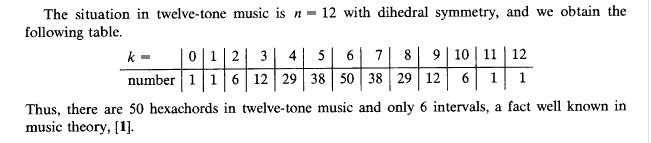Amer. Math. Monthly, 92:51 – 54, 1985

But can we go still further, by equivalencing something other than just rotations and reflection? Can we get it down to - say - 200?